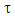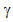## Power Law Constants (n and K) Calculation

Many types of drilling mud are classified as non-Newtonian fluid which is the behavior between Newtonian fluid model and Bingham Plastic model. The relationship between shear rate and shear stress is defined by the power law model shown below:Where= shear rate

K = consistency factor= shear rate

n = flow behavior index